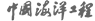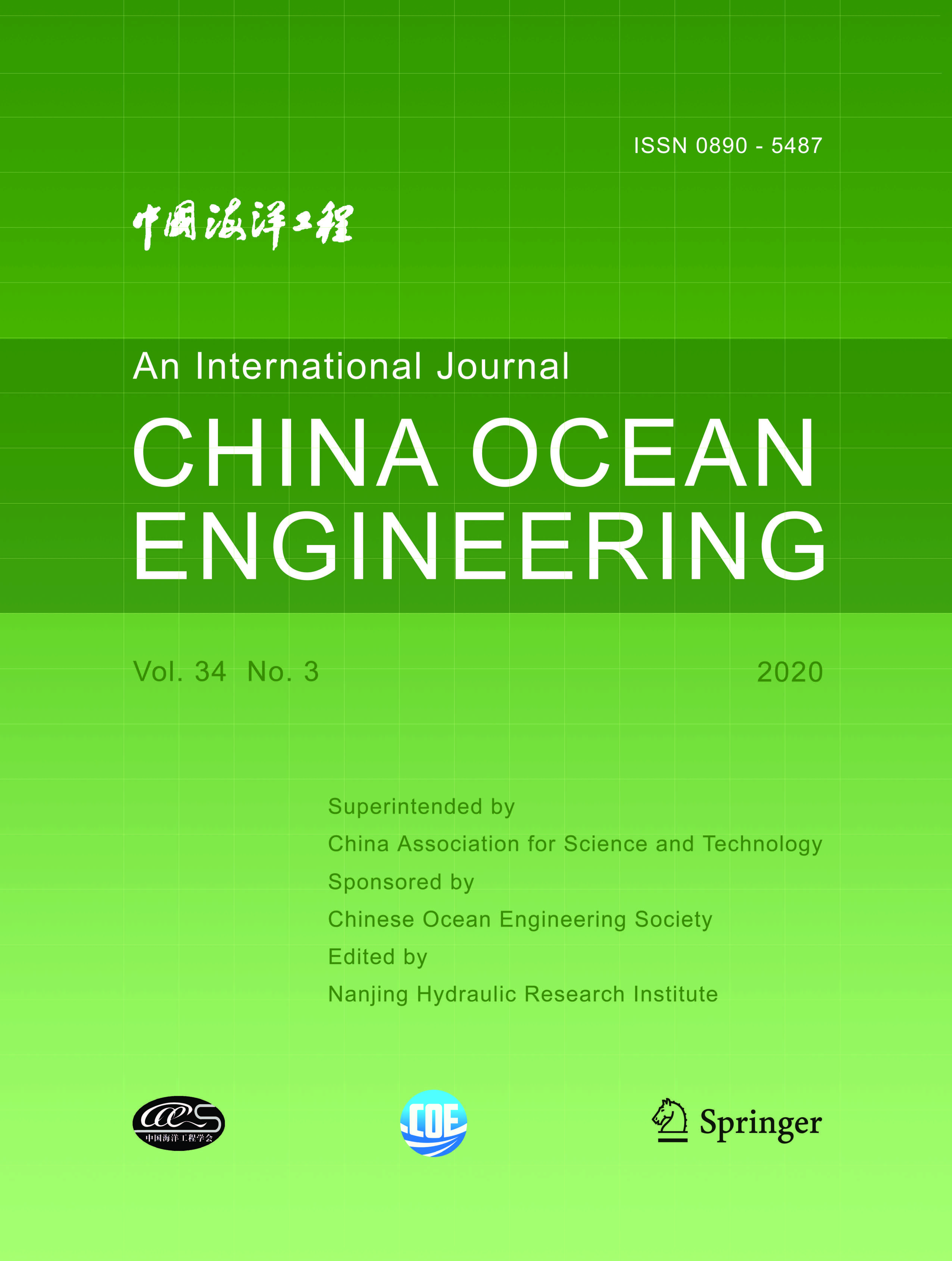ISSN  0890-5487 CN 32-1441/P

## 2001 Vol.(1)

Display Mode：          |

2001, (1): -.
[Abstract](341)
Abstract:
In this paper, the characteristics of different forms of mild slope equations for non-linear wave are analyzed, and new non-linear theoretic models for wave propagation are presented, with non-linear terms added to the mild slope equations for non-stationary linear waves and dissipative effects considered. Numerical simulation models are developed of non-linear wave propagation for waters of mildly varying topography with complicated boundary, and the effects are studied of different non-linear corrections on calculation results of extended mild slope equations. Systematical numerical simulation tests show that the present models can effectively reflect non-linear effects.
2001, (1): -.
[Abstract](337)
Abstract:
A time-domain method is applied to simulate nonlinear wave diffraction around a surface piercing 3-D arbitrary body. The method involves the application of Taylor series expansions and the use of perturbation procedure to establish the corresponding boundary value problems with respect to a time-independent fluid domain. A boundary element method based on B-spline expansion is used to calculate the wave field at each time step, and the free surface boundary condition is satisfied to the second order of wave steepness by a numerical integration in time. An artificial damping layer is adopted on the free surface for the removal of wave reflection from the outer boundary. As an illustration, the method is used to compute the second-order wave forces and run-up on a surface-piercing circular cylinder. The present method is found to be accurate, computationally efficient, and numerically stable.
2001, (1): -.
[Abstract](503)
Abstract:
Statistical properties of winds near the Taichung Harbour are investigated. The 26 years'incomplete data of wind speeds, measured on an hourly basis, are used as reference. The possibility of imputation using simulated results of the Auto-Regressive (AR), Moving-Average (MA), and/ or Auto-Regressive and Moving-Average (ARMA) models is studied. Predictions of the 25-year extreme wind speeds based upon the augmented data are compared with the original series. Based upon the results, predictions of the 50- and 100-year extreme wind speeds are then made.
2001, (1): -.
[Abstract](321)
Abstract:
An obvious motivation of this paper is to examine the effectiveness of the lateral vibration control of a jacket type offshore platform with an AMD control device, in conjunction with H2 control algorithm, which is an optimal frequency domain control method based on minimization of H2 norm of the system transfer function. In this study, the offshore platform is modeled numerically by use of the finite element method, instead of a lumped mass model. This structural model is later simplified to be single-degree-of-freedom (SDOF) system by extracting the first vibration mode of the structure. The corresponding "generalized" wave force is determined based on an analytical approximation of the first mode shape function, the physical wave loading being calculated from the linearized Morison equation. This approach facilitates the filter design for the generalized force. Furthermore, the present paper also intends to make numerical comparison between H2 active control and the corresponding passive control using a TMD with the same device parameters.
2001, (1): -.
[Abstract](340)
Abstract:
Mooring model experiment and mooring line tension determination are of significance to the design of mooring systems and berthing structures. This paper mainly involves: (a) description and analysis of a mooring model experiment; (b) derivation of static equilibrium equations for a moored ship subjected to wind, current and waves; (c) solution of mooring equations with the Monte Carlo method; (d) qualitative analysis of effects of pier piles on mooring line forces. Special emphasis is placed on the derivation of static equilibrium equations, solution method and the mooring model experiment.
2001, (1): -.
[Abstract](328)
Abstract:
A new expression for calculating suspended fine-sediment deposition rate is developed based on theoretic analysis and experiments. The resulting equation is applied to simulation of fine sediment deposition in the reclaimed land in the Hangzhou Bay. China. The hydrodynamic environment in this area is solved by use of a long wave model, which gives the 2D-velocity field and considers bathymelric changes due to fine sediment deposition. The expression is proved convenient to use in engineering practice, and the predicted deposition rate agrees with the annual data available from field measurements from the first year to the third year after the construction of the long groin as a reclaiming method.
2001, (1): -.
[Abstract](331)
Abstract:
2001, (1): -.
[Abstract](342)
Abstract:
For evaluation of the permanent deformation of a sea embankment under stochastic earthquake excitation, a robust dynamic risk analytical method is presented based on conventional permanent deformation analysis and stochastic seismic response analysis. This method can predict not only the mean value of maximum permanent deformation but also the reliability corresponding to different deformation control standards. The earthquake motion is modelled as a stationary Gaussian filtered white noise random process. The predicted average maximum horizontal permanent displacement is in agreement with the conventional result. Further studied are the reliability of permanent deformation due to stochastic wave details at one seismic motion level and the risk of permanent deformation due to stochastic seismic strength, i. e., the maximum acceleration in a long period. Therefore, it is possible to make the optimal design in terms of safety and economy according to the importance of a sea embankment. It is suggested that the improved stochastic seismic model that can catch the behavior of the non-stationary random process for sea embankments should be further studied in future.
2001, (1): -.
[Abstract](329)
Abstract:
The motion of particle clouds formed by dumping dredged material into quiescent waters is experimentally and numerically studied. In the numerical model, the particle phase is modeled by the dispersion model, and turbulence is calculated by the large eddy simulation. The governing equations, including the filtered Navier-Stokes equations and mass transport equation, are solved based on the operator-splitting algorithm and an implicit cubic spline interpolation scheme. The eddy viscosity is evaluated by the modified Smagorinsky model including the buoyancy term. Comparisons of main flow characteristics, including shape, size, average density excess, moving speed and the amount of particles deposited on the bed, between experimental and computational results show that the numerical model well predicts the motion of the cloud from the falling to spreading stage. The effects of silt-fence on the motion of the particle cloud are also investigated.
2001, (1): -.
[Abstract](393)
Abstract:
The scour of the seabed under a pipeline is studied experimentally in this paper. Tests are carried out in a U-shaped oscillatory water tunnel with a box imbedded in the bottom of the test section. By use of the standard sand, clay and plastic grain as the seabed material, the influence of the bed material on the scour is studied. The relationship between the critical initial gap-to-diameter ratio above which no scour occurs and the parameters of the oscillating flow is obtained. The self-burial phenomenon, which occurs for the pipeline not fixed to two sidewalls of the test section, is not observed for the fixed pipeline. The effect of the pipe on sand wave formation is discussed. The maximum equilibrium scour depths for different initial gap-to-diameter ratios, different Kc numbers and different bed sands are also given in this paper.
2001, (1): -.
[Abstract](345)
Abstract:
For prediction of the extreme significant wave height in the ocean areas where long term wave data are not available, the empirical method of extrapolating short term data (1-3 years) is used in design practice. In this paper two methods are proposed to predict extreme significant wave height based on short-term daily maxima. According to the da-a recorded by the Oceanographic Station of Liaodong Bay at the Bohai Sea, it is supposed that daily maximum wave heights are statistically independent. The data show that daily maximum wave heights obey log-normal distribution, and that the numbers of daily maxima vary from year to year, obeying binomial distribution. Based on these statistical characteristics, the binomial-log-normal compound extremum distribution is derived for prediction of extreme significant wave heights (50-100 years). For examination of its accuracy and validity, the prediction of extreme wave heights is based on 12 years' data at this station, and based on each 3 years' data respectively. The results show that with consideration of confidence intervals, the predicted wave heights based on 3 years' data are very close to those based on 12 years' data. The observed data in some ocean areas in the Atlantic Ocean and the North Sea show it is not correct to assume that daily maximum wave heights are statistically independent; they are subject to Markov chain condition, obeying log-normal distribution. In this paper an analytical method is derived to predict extreme wave heights in these cases. A comparison of the computations shows that the difference between the extreme wave heights based on the assumption that daily maxima are statistically independent and that they are subject to Markov Chain condition is smaller than 10%.
2001, (1): -.
[Abstract](323)
Abstract:
Two- and three-dimensional finite element analysis programs for pile-soil interaction are compiled. Duncan-Chang's Model is used. The construction sequence of the pier is modeled. The pile-soil interface element is used. The influence of the combination type of piles on the deformation of bank slope and pile behaviour is analyzed. Different designs of a pile-supported pier are compared thoroughly. Calculation results show that the stresses and displacements of the pile are directly related to the distance from the bank slope and the direction of inclination. An inclined prop pile set in the rear platform would remarkably reduce the stresses of piles and the displacement of the pier.
2001, (1): -.
[Abstract](320)
Abstract:
The abandoned Yellow River Delta coast is a typical erodible silty and muddy coast in China. The paper analyses the marine dynamic characteristics and the mechanism of beach erosion of this area. Analysis and calculation show that in this sea area wave and tidal current action should be considered. Based on the above analysis, an equilibrium beach profile calculation model is developed, in which the wave-current interaction is considered while sediment supply and sediment re-deposition are neglected. The model consists of four parts: (1) calculation of wave parameters, (2) calculation of velocity due to wave-current interaction at different water depth, (3) calculation of friction velocity and shear stress at different water depths, and (4) calculation of the amount of sediment erosion, erosion intensity and variation of beach profile. Calculated results are in good agreement with observed data. Finally, the evolution tendency is discussed and the equilibrium beach profile of this coast is calculated. Based on prediction, the slope of equilibrium beach profile is about 1/85.
2001, (1): -.
[Abstract](326)
Abstract:
Based on the time dependent mild slope equation including the effect of wave energy dissipation, an expression for the energy dissipation factor is derived in conjunction with the wave energy balance equation, and then a practical method for the simulation of wave height and wave set-up in nearshore regions is presented. The variation of the complex wave amplitude is numerically simulated by use of the parabolic mild slope equation including the effect of wave energy dissipation due to wave breaking. The components of wave radiation stress are calculated subsequently by new expressions for them according to the obtained complex wave amplitude, and then the depth-averaged equation is applied to the calculation of wave set-up due to wave breaking. Numerical results are in good agreement with experimental data, showing that the expression for the energy dissipation factor is reasonable and that the new method is effective for the simulation of wave set-up due to wave breaking in nearshore regions.

### Current Issue1. Editor-in-Chief:

2. Superintended by:

CHINA ASSOCIATION FOR SCIENCE AND TECHNOLOGY

Chinese Ocean Engineering Society （COES）

4. Edited by:

Nanjing Hydraulic Research InstituteAddress: 34 Hujuguan, Nanjing 210024, China Pos: 210024 Tel: 025-85829388 E-mail: coe@nhri.cn

Support by Beijing Renhe Information Technology Co. Ltd E-mail: info@rhhz.net